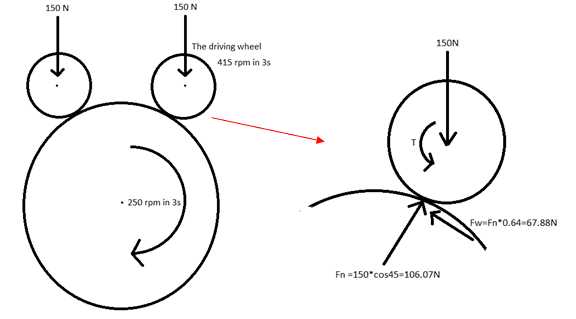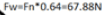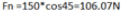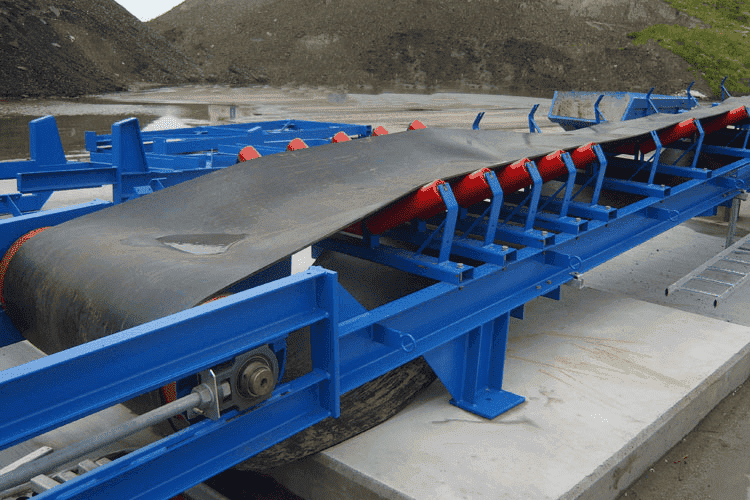# Rotation of a roll by a driving wheel

• Rusjar

#### Rusjar

The bigger circle is a hollow cylinder (steel) with a length of 0.6m and a diameter of 0.133m and a mass of 8kg. The 2 smaller circles are rubber wheels with a diameter of 0.080 and mass of 0.2kg. Both the roll and the wheels have ball bearings and are mounted on a shaft. On both the shafts of the wheels a force of 150N is pressed down. The shaft of the right wheel is connected directly to a motor.
If I want the roll to accelerate to a speed of 250rpm in 3 seconds, what is the torque required for the driving wheel? I’ve made a calculation myself but I’m not sure if its correct.I = 0.5*0.2*0.0042 0.04^2 = 0.00016 kgm2
415 rpm = 43.46 rad/s = ω(3s)

Now: ω(3s) = α * t
43.46 = α * 3

T = I * α
T – 67.88*0.04 = 0.00016 * 14.49
T = 2.7 Nm

Is this calculation correct? Is there 2.7 Nm of torque required in order for the roll to rotate with 250 rpm in 3 seconds?

Last edited:
•Delta2

You haven't fully specified the geometry of the roller--missing inside diameter. So there's that. If this is intended to be a real device then it is not frictionless (rolling resistance), so that will rob some energy (which you may want to ignore for a first-order analysis). Of which, perhaps an energy/power analysis will produce a usable result.

Summary: I want to rotate a roll by using a smaller rubber wheel.

The bigger circle is a hollow cylinder (steel) with a length of 0.6m and a diameter of 0.133m and a mass of 8kg. The 2 smaller circles are rubber wheels with a diameter of 0.080 and mass of 0.2kg.
[...]
I = 0.5*0.2*0.042 = 0.00016 kgm2
Can you justify this calculation? The moment of inertia of a hoop of radius r should be I=mr2I=mr2. For the cylinder, m = 8 kg, and r = 0.6 mr=0.067m That comes to 8 times 0.360.004489 = 0.035912 kg m^2. The result you give is smaller.

You have not accounted for the torque required to accelerate either of the smaller wheels.

The systematic approach is to start with some algebra. Write down equations for the angular acceleration of each of the three wheels using Newton's law for angular acceleration: ##\sum \tau=I \alpha##. Make a list of the torques on each wheel so that you can fill in the ##\sum \tau## part. Write down equations relating the torques to each other using Newton's third law for the force of each paired wheel on the other member of its pair. Write down equations relating the angular accelerations to each other using the gear ratios.

[There is considerable simplification available -- one could decide to treat each of the three wheels as having an effective moment of inertia relative to the rotation rate of one particular wheel and eliminate a bunch of equations all at once]

Given the desired angular acceleration of the large wheel you should be able to come up with enough simultaneous equations to solve for the unknown torque on the smaller wheel.

Hello Rusjar,!
bigger circle is a hollow cylinder
So its momentum of inertia is ##I = mr^2##, some 0.035 kgm2

0.5*0.2*0.042 = 0.00016
I get 0.0042, but you meant 0.5*0.2*0.042.

So for the two rubber wheels you need 14.5 * 0.00016 * 2 = 0.0046 N##\times ##m

Main question: what's the
67.88*0.04
that comes out of the blue ?

I would think you need the cylinder to accelerate with 8.7 rad/s2, so you need 0.31 N##\times ##m in addition to the little bit you need for the rubber wheels.

Boy, do I type slow ! @jbriggs444 and @lewando were a lot quicker !

Last edited:
r = 0.6 m
No, r is only 6.65 cm. But you do type fast !•jbriggs444
Hello Rusjar,!
So its momentum of inertia is ##I = mr^2##, some 0.035 kgm2

I get 0.0042, but you meant 0.5*0.2*0.042.

So for the two rubber wheels you need 14.5 * 0.00016 * 2 = 0.0046 N##\times ##m

Main question: what's the
that comes out of the blue ?

I would think you need the cylinder to acceleratee with 8.7 rad/s2, so you need 0.31 N##\times ##m in addition to little bit you need for the rubber wheels.

Boy, do I type slow ! @jbriggs444 and @lewando were a lot quicker !
I'm sorry, the 0.0042 should be 0.004 0.04^2.
The 67.88*0.04 is the moment created by the friction force. That one should be included right?
And I calculated the torque for the wheel, and the wheel is not hollow thus should be I = 0.5*m*r^2

the 0.0042 should be 0.004.
No. it should be 0.042

friction force
Explain. In the small print I seeandbut I have no idea where they come from. Do you ?

That one should be included right?
No. You can simply add required torques to find the total torque needed.
Friction needs to be enough to start without slipping if that is desired. Certainly not maximum.

Compare: pushing a car goes with F = ma . You don't even look at the friction.

No. it should be 0.042

Explain. In the small print I see View attachment 251386 and View attachment 251387 but I have no idea where they come from. Do you ?

No. You can simply add required torques to find the total torque needed.
Friction needs to be enough to start without slipping if that is desired. Certainly not maximum.

Compare: pushing a car goes with F = ma . You don't even look at the friction.
The Fw and Fn forces are coming from the 150N which is pushing down on the roll. These are the reaction forces.

I cannot agree with you on that last sentence. If you would push a car, the friction forces on the wheels play a major role in how much force you would need.

the friction forces on the wheels play a major role in how much force you would need.
Yes, but that's the rolling friction, not the max braking friction.

You haven't explained theandyet ...

Yes, but that's the rolling friction, not the max braking friction.

You haven't explained the View attachment 251389 and View attachment 251390 yet ...
Well if the shaft through the wheel exerts a force of 150N downwards, then there will be a normal force (Fn) at the place where the wheel makes contact with the roll, right? And that normal force multiplied with the friciton coefficient of rubber and steel gives a friction force. Or is this not correct?

gives a friction force
No. It gives the maximum friction force that can be exerted. That's why I compared with pushing a car -- unwise to try it with the brakes on.

Note that to accelerate the left wheel, a torque of 0.00233 is needed, so a (friction) force of only 0.06 N.

For the hollow cylinder alone a friction force of 4.64 N.

Altogether 4.75 N on the rim of the driving wheel -- but of course you use a motor on its driving axis.

And to be increased with what you loose to rolling friction

No. It gives the maximum friction force that can be exerted. That's why I compared with pushing a car -- unwise to try it with the brakes on.

Note that to accelerate the left wheel, a torque of 0.00233 is needed, so a (friction) force of only 0.06 N.

For the hollow cylinder alone a friction force of 4.64 N.

Altogether 4.75 N on the rim of the driving wheel -- but of course you use a motor on its driving axis.

And to be increased with what you loose to rolling friction
I understand the part of the friction now, thanks!

But it's still not clear to me how I can calculate the required torque (for the motor connected to the wheel) so that the roll will rotate with 250 rpm and the wheel will rotate with 415 rpm when a force of 150N pushing downwards on the shaft of the wheel.

Like with the wheel, the downward force on the hollow cylinder is compensated for by its bearing (it is being supported, right ?) and does not do anything to make it rotate, i.e. exerts no torque.

Like with the wheel, the downward force on the hollow cylinder is compensated for by its bearing (it is being supported, right ?) and does not do anything to make it rotate, i.e. exerts no torque.
So I can just calculate T = I * a (alfa) for the driving wheel on the right without having to deal with the 150N downwards?

Correct.

re friction:
How is the hollow cylinder supported ? Is there a reason for the 150 N ?

Correct.

re friction:
How is the hollow cylinder supported ? Is there a reason for the 150 N ?
The hollow cylinder contains 2 bearings on each end and has a shaft going through it. The shaft is fixed on both ends.

The 150N is caused by the weight of a system where the two wheels are a part of.

I would expect the friction due to the 300 N in total requires more torque than you need to overcome the rotational inertia. But I don't think there's a good method to quantify that friction, other than by trial and error (aka experimentation).

If I understand this right, the hollow cylinder has to bear the weight of the contraption above it. On what underground ? Desert sand ? Or glass plate ?

Does the lot have to balance on this one single cylinder ?

Are we discussing a detail without any knowledge of the surroundings ?

The 150N is caused by the weight of a system where the two wheels are a part of.
One would expect that the weighted structure would also prevent the drive wheel and the idler wheel from spreading. You have not shown the resulting force on the free body diagram.

I would expect the friction due to the 300 N in total requires more torque than you need to overcome the rotational inertia. But I don't think there's a good method to quantify that friction, other than by trial and error (aka experimentation).

If I understand this right, the hollow cylinder has to bear the weight of the contraption above it. On what underground ? Desert sand ? Or glass plate ?

Does the lot have to balance on this one single cylinder ?

Are we discussing a detail without any knowledge of the surroundings ?
I want to make a system which rides between the conveyor belt and the rollers underneath.
The system is balanced because it is fixed to the conveyor belt, it only leans on the rolls and rotates them.fixed to the conveyor belt
?

What is the purpose of this system ?

The check the condition of the bearings in the rolls, by rotating them an ultrasound can be detected.

Doesn't the belt itself rotate the rollers ?

No, the belt doesn't move while the systems is going over the rollers. So the weight of the belt is leaning on the system, that's where the 150N comes from.

Yeah, after a cup of coffee (actually, two) that insight dawned on me. So it's kind of a mobile system for troubleshooting the roller bearings whilst the conveyor is not operating. The 100 m/s is for the ultrasound.

Sure hope the belt is not a few kilometers long.

A compliment: I think you presented the situation quite well ( so my #17 is a bit too critical ). Best of luck and my guess is the friction in the bearings can't be ignored wrt the inertia. You could experiment with an electric hand drill ...

It's indeed a mobile system.

I was hoping for an easy way to calculate the required torque but I know now I still have some work to do. Thanks for the answers though! Experimenting with an electric hand drill is a very good one, haven't thought about that, thanks ;)